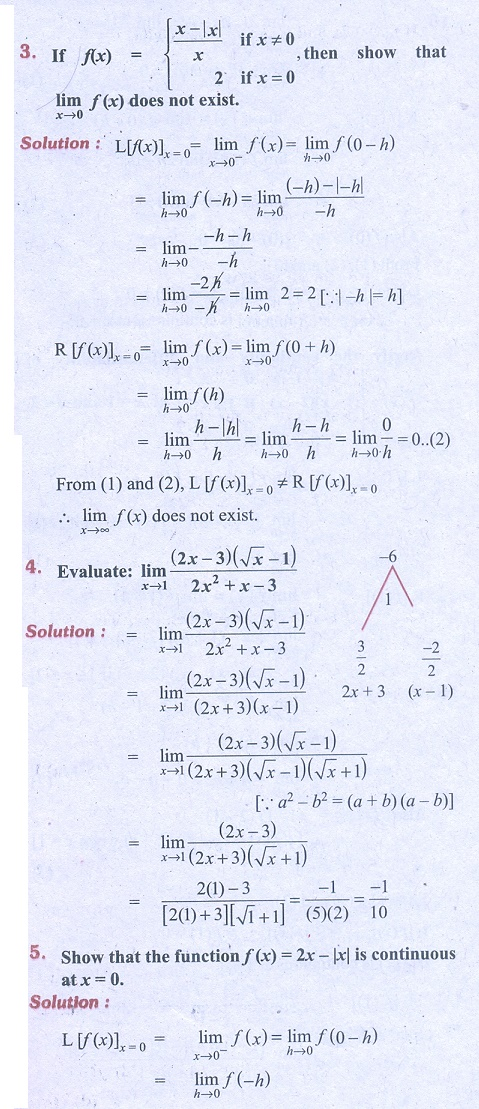Home | | Business Maths 11th std | Differential Calculus: Miscellaneous Problems

# Differential Calculus: Miscellaneous Problems

Business Mathematics and Statistics Book back answers and solution for Exercise questions - Differential Calculus: Miscellaneous ProblemsTags : Problem Questions with Answer, Solution | Mathematics , 11th Business Mathematics and Statistics(EMS) : Chapter 5 : Differential Calculus
Study Material, Lecturing Notes, Assignment, Reference, Wiki description explanation, brief detail
11th Business Mathematics and Statistics(EMS) : Chapter 5 : Differential Calculus : Differential Calculus: Miscellaneous Problems | Problem Questions with Answer, Solution | Mathematics